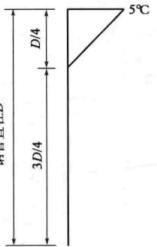How Sofistik interpret nonliear temperature on circular section?

I have a circular section with D=1000 mm The nonlinear temperature is shown in the picI assume that the nonlinear temperature on the section will be like the red portion in the picI can calculate the equivalent axial force and moment based on the equation in Sofiload manual:

Hand calculation:

Axial force N=2061.7kN, moment=557.8kNxm

While Sofistik give me,
Axial force N=793.3kN, moment=313.2kNxm.

Teddy dats are attached.
Nonl Temp on Circular Section.dat (83 Bytes)
Aqua.dat (252 Bytes)
SofimshC.dat (175 Bytes)